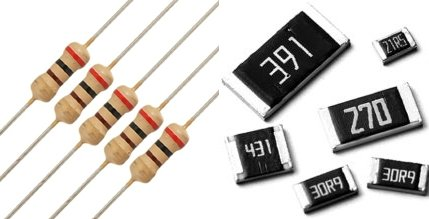# All About Resistor / How To Read Code Of SMD Resistor / Colour Code Of Resistor

Introduction .

Resistor is most common passive two terminal component used in electronics circuit . This device used in various circuits. Without resistor we can’t imagine any circuit . Resistor is very important device to make a circuit. Any simple to simple circuit we can see made using resistor.

## Working Of Resistor

The main function of resistor is limits the flow of current. It restricts and controls the power supply between other components. Controlling of supply through the path because it make disturbance in flow of electrons. The resistance of any resistor is noted by ohm (Ω), ohm (symbol of ohm is omega) is unit of measurement of resistance of any resistor. If a resistor has large value of resistance then it creates more disturbance to flow of electron . It means large value resistor have large resistance and small value resistor creates very little disturbance and it has small resistance.

Symbol.

### Types of Resistors     Must Read Method Of Ceramic Capacitor Code Reading

• Fixed resistors:  This type of resistors have fixed value. They are used in electronics circuits where does not require to changing in value of resistance . This set a fixed conditions in a circuit. Values of these resistors  are determined during the designing of the circuit, and they should never need to be changed to "adjust" the circuit.
• Variable resistors:   These resistors used in adjustable value of Electric Resistance as required . This value can be changed manually. These consist of a fixed resistor element and a slider which taps onto the main resistor element. This gives three connections to the component, where two connected to the fixed element, and the third is the slider or movable. In this way the component acts as a variable potential divider if all three connections are used. It is also to connect to the slider and only one end to provide a resistor with variable resistance.
##### Fixed resistor types

There are mainely 6 types of fixed resistor:

• Carbon composition:  . They are formed by mixing of carbon granules with a binder which was then made into a small rod. This type of resistor was large by today's standards and suffered from a large negative temperature coefficient. The resistors also suffered from a large and erratic irreversible changes in resistance as a result of heat or age. In addition to this the granular nature of the carbon and binder lead to high levels of noise being generated when current flowed.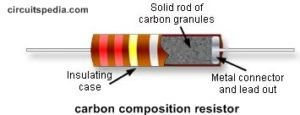• Carbon film:   The carbon film resistor is formed using a ceramic carrier rod onto which a thin layer of pure carbon is deposited as a film. It is the thin carbon film that acts as the resistive element. To enable the carbon film resistor to have the correct resistance, a helical cut is normally made into the film. This increases the length of the path and also reduces the width of the resistive element .                                                                                  This type resistor is formed by "cracking" a hydrocarbon onto a ceramic former and then deposited film had its resistance set by cutting a helix into the film. These resistors used in many RF applications. They exhibited a temperature coefficient of between -100 and -900 ppm / °Celcius. The carbon film is protected by a conformal epoxy coating or a ceramic tube.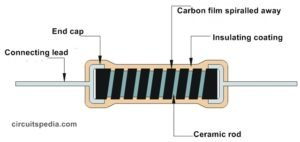The carbon film resistor provides better performance in many respects than the carbon compositing resistor, but this resistor type was overtaken by metal oxide film and metal film resistors which gave even better levels of performance.
• Metal oxide film:   This resistor uses a metal oxide film deposited on a ceramic rod. Metals oxide such as tin oxide are deposited onto the ceramic rod. This type of resistor has a temperature coefficient of around ±15 parts per million / °K, giving it a far superior performance to that of any carbon based resistor. This type of resistor is now one of the most widely used form of resistor along with the metal film type rather than using a carbon film. The resistance of the component is adjusted in two ways. First the thickness of the deposited layer is controlled during the initial manufacturing stages. Then it can be more accurately adjusted by cutting a helical grove in the film. Again the film is protected using a conformal epoxy coating. Noise level of this resistors have lower than carbon film resistor. This type of resistor can be supplied with much closer tolerance of ±5%, ±2% being standard, and with ±1%.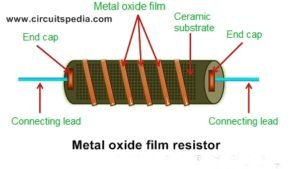• Metal film:  The metal film resistor construction is similar to the carbon film resistor except that the material used for constructing the film is different. This type of resistor uses a metal film. Metals such as nickel alloy may be used. In carbon film resistors, carbon is used to construct the film whereas in metal film resistors, tin and antimony or nickel chromium is used to construct the film. In metal film resistor, the desired resistance value can be achieved by either trimming the thickness of metal layer or by cutting the metal film in a helical manner along its length. This is generally done by using the lasers. Once the desired resistance value is obtained, the cutting of metal is stopped. The metal film resistors is very similar to the metal oxide film resistor in terms of visual appearance and performance. Resistance of metal film resistor depends on the thickness of metal film layer and width of helical metal film cut.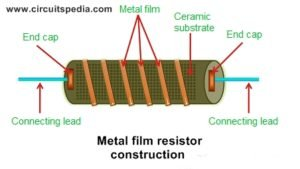• Wire wound:  A wire wound resistor is a resistor where a wire with a high resistivity is wrapped around an insulating core to provide the resistance. The resistance value is dependent on the resistivity of the wire, the cross section and the length. Since these parameters can be accurately controlled, a high precision can be achieved. For high tolerance requirements, the resistance value is measured to determine exactly the cut to length of the wire. To create a high resistance, the wire diameter needs to be very small and the length very long. Therefore wire wound resistors are mainly produced for lower resistance values. For low power ratings, very thin wire is used. The handling of the wire is for this matter critical. Any damage may sever contact. After winding the wire is well protected. These resistors have a quite different construction. Compared to other resistor types as the metal film, the wire diameter is relatively big and therefore more robust.

This resistor type is generally used in high power applications.. The more expensive varieties are wound on a ceramic former and they may be covered by a vitreous or silicone enamel. This resistor type is suited to high powers and exhibits a high level of reliability at high powers along with a comparatively low level of temperature coefficient, although this will depend on a number of factors including the former, wire used, etc.

Thin film:   Thin film technology is used for most of the surface mount types of resistor. As these are used in their billions these days, this makes this form of resistor technology one of the most widely used.

Termination and mounting

Through-hole resistors come with legs which easily entered through hole on the pcb board. Both terminals have legs of specific length which is used during connecting with other components using solder. This type of resistors are in axial size and take more space on circuit implementation. The size of an axial resistor is relative to its power rating. A common ½W resistor measures about 9.2mm across, while a smaller ¼W resistor is about 6.3mm long.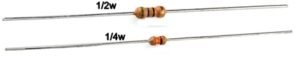### Must Read Method Of Ceramic Capacitor Code Reading

Surface mount (non leaded)

Surface mount resistor are mainly tiny size and rectangular shape in black colour. Terminal on its  side with smaller, shiny, silver, with conductive edges. These resistors are intended to sit on top of PCBs, where they’re soldered onto mating landing pads. Because these resistors are so small, they’re usually set into place by a robot, and sent through an oven where solder melts and holds them in place. SMD resistors come in standardized sizes; usually either 0805 (0.8mm long by 0.5mm wide), 0603, or 0402. They’re great for mass circuit-board-production, or in designs where space is a precious commodity. They take a steady, precise hand to manually solder.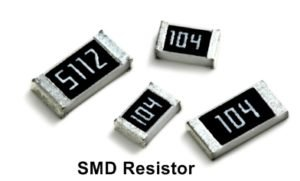Surface Mount Resistors are printed with either a 3 or 4-digit numerical code which is similar to that used on the more common axial type resistors to denote their resistive value. Standard SMD resistors are marked with a three-digit code, in which the first two digits represent the first two numbers of the resistance value with the third digit being the multiplier, either x1, x10, x100 etc. For example

103 = 10 × 1,000 ohms = 10 kiloΩ

392 = 39 × 100 ohms = 3.9 kiloΩ

563 = 56 × 1,000 ohms = 56 kiloΩ

105 = 10 × 100,000 ohms = 1 MegaΩ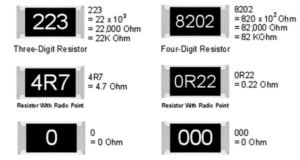Surface mount resistors that have a value of less than 100Ω’s are usually written as: “390”, “470”, “560” with the final zero representing a 10 xo multiplier, which is equivalent to 1. For example:

390 = 39 × 1Ω = 39Ω or 39RΩ

470 = 47 × 1Ω = 47Ω or 47RΩ

###### How to read colour code of resistor            Must Read What is 555 Timer

Method of finding the value of through hole resistor

•  Digit digit multiplier tolerance (for 4 band resistor )

Digit digit digit multiplier tolerance (for 5 band resistor)

Yellow Violet Red = 4 7 2 = 4 7 x 102 = 4700Ω or 4.7or 4k7.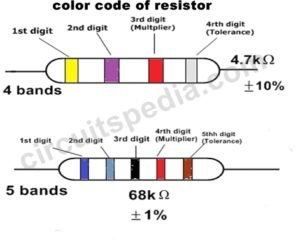Green Grey Black Red Brown=6 8 0 2 = 680x102 =68000or 68kΩ

##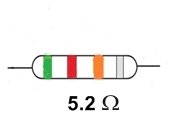If A resistor colored  Green-Red-Gold-Silver  then its value is 5.2 Ω and tolerance of +/- 10%.

A resistor colored Brown-Green-Grey-Silver-Red would be 1.58 Ω with a tolerance of +/- 2%.

Colour Code Table of Resistor

### Must Read Method Of Ceramic Capacitor Code Reading

Series connection

A series circuit is a circuit in which resistors are arranged in a chain, so the current has only one path to take. The current is the same through each resistor. The total resistance of the circuit is found by simply adding up the resistance values of the individual resistors:

equivalent resistance of resistors in series : R = R1 + R2 + R3 + ....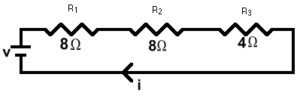series circuit is shown in the diagram above. The current flows through each resistor in turn. If the values of the three resistors are:

R1=8 Ω , R2= 8 Ω , R3=4Ω then  total resistance = 8+8+4= 20 Ω

With a 10 V battery, by V = I R the total current in the circuit is:

I = V / R = 10 / 20 = 0.5 A. The current through each resistor would be 0.5 A

Parallel connection

A parallel circuit is a circuit in which the resistors are arranged with their heads connected together, and their tails connected together. The current in a parallel circuit breaks up, with some flowing along each parallel branch and re-combining when the branches meet again. The voltage across each resistor in parallel is the same.

The total resistance of a set of resistors in parallel is found by adding up the reciprocals of the resistance values, and then taking the reciprocal of the total:

equivalent resistance of resistors in parallel: 1 /R = 1 / R1 + 1 / R2 + 1 / R3 +....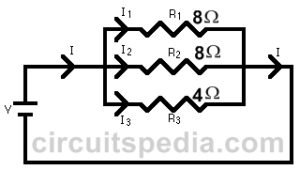A parallel circuit is shown in the diagram above. In this case the current supplied by the battery splits up, and the amount going through each resistor depends on the resistance. If the values of the three resistors are:

R1=8 Ω , R2= 8 Ω , R3=4Ω then  total resistance =1/R=1/8+1/8+1/4

1/R=1/2, then R=2 Ω

With a 10 V battery, by V = I R the total current in the circuit is: I = V / R = 10 / 2 = 5 A.

Must Read Touch Switch For ON-OFF Light/Fan